• Call Now

1800-102-2727•

# Resultant Force: Definition, Concepts and Examples

If someone asks you to walk on a tightrope, will you do it? Most certainly you will not. Because you will not be able to balance yourself on thin rope and you might fall. Look at the tightrope walker in the picture below, he is balancing all the forces with the help of a rod. In this article we will study about resultant force, balanced force and unbalanced force in detail.## Resultant Force

The vector sum of all the external forces that are acting on a system represents the resultant force on that object. Resultant force is also called Net Force.

The summation of all the forces gives the value of the net force acting on the body, and the vector provides the direction in which the resultant will be effective.

As shown in the figure, various forces are acting on the body with different magnitudes as well as in different directions, hence the resultant will give the overall effect.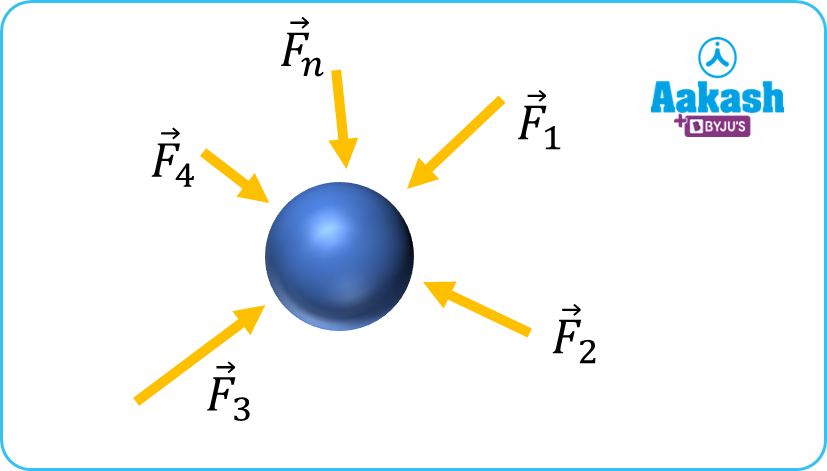From figure,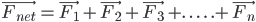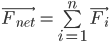## Balanced Force

If the resultant force of all the forces acting on a body sums up to zero, then the forces acting on the body are known as balanced forces. Let us look at some examples of balanced forces and understand how the body’s state of motion remains unaffected

Mathematically,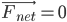You must have played a tug-of-war game. If the game is between two equally matched teams. Neither of the teams is able to pull the opposing team towards itself. This is an example of balanced forces.

## Unbalanced Force

When the resultant force acting on a body is not equal to zero, the forces acting on the body are known as unbalanced forces. The body acted upon by unbalanced forces changes its state of motion. Let us look at some examples of unbalanced forces to understand their nature better.

Mathematically,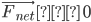We see children playing seesaw in the park. If their weights are different then we see that heavier child is at the bottom and lighter one goes to top. When the child at bottom pushes the ground by his/her feet that again creates unbalancing and he/she goes up. This motion keeps repeating because of unbalanced forces acting on the seesaw.

## Translational Equilibrium

A body is in translational equilibrium when it is at rest or moving with constant velocity in an inertial frame of reference.

When the net/resultant force on an object is zero, then the object is said to be in a state of translational equilibrium.

In other words, we can say that if only balanced forces are acting the body then it is under translational equilibrium.

Mathematically,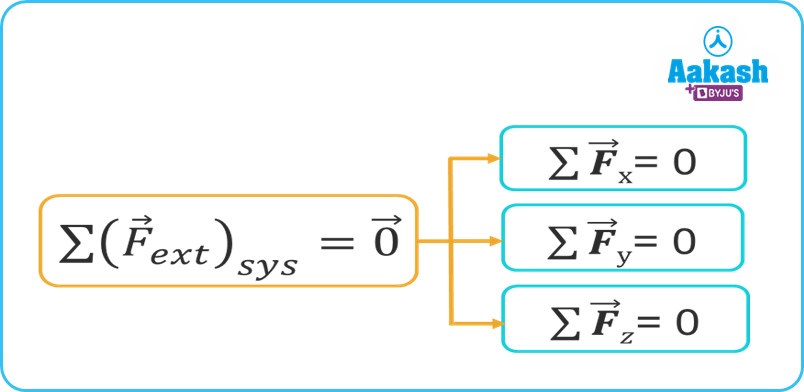Equations on the right side are given in component form in x, y, z directions. That we use extensively while solving problems.

Video Explanation:

## Practice Problems of Resultant Force

Q1. Three blocks are suspended by a light string as shown in the given figure. What is the value of T3  so that the system is in equilibrium? (Take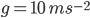)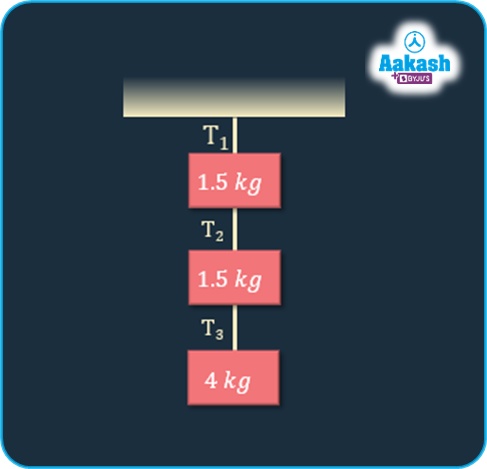Solution: Let’s consider 4 Kg blocks and show all forces acting on them. Then apply equilibrium condition,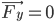.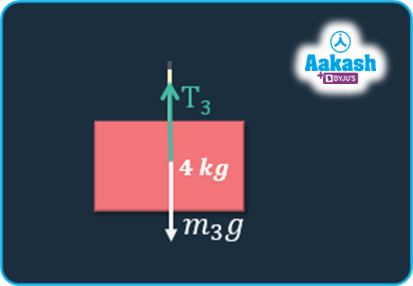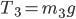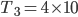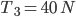Q2. Three blocks are suspended by a light string as shown in the given figure. What is the value of T1  so that the system is in equilibrium? (Take)Solution:

Let’s consider each block one by one and show all forces acting on them. Then apply equilibrium condition ,.

Lower Block:Middle Block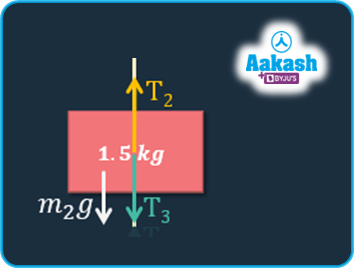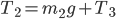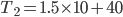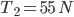Upper Block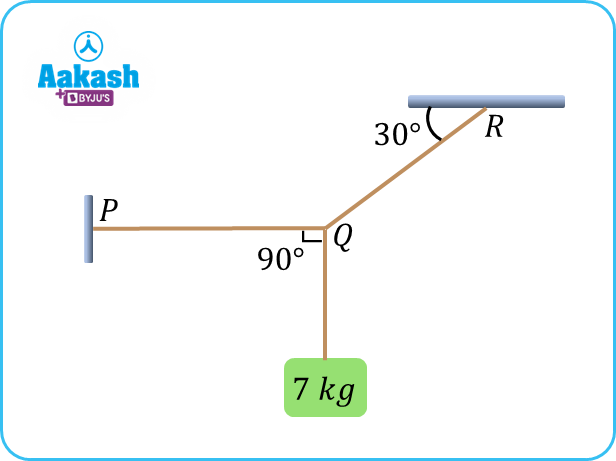Q3. Find the tension in horizontal string PQ and the string QR shown in the figure? ()

Solution:

Let’s assume tension in the string and show all forces, shown in the figure below

From the figure we get,

For equilibrium in y direction,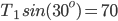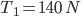For equilibrium in x direction,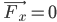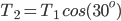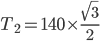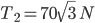Q4. Two blocks are connected to a string and supported by a pulley as shown in the figure. Find the value of ‘m’ such that both the blocks are in equilibrium. ()Solution:

To get the values of the mass ‘m’, draw the FBD of the whole system, then apply the equilibrium conditions on the resolved force components.

For mass m,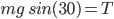. . . . . . . (1)

For mass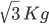,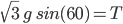. . . . . . .(2)

Equating (1) and (2) we get,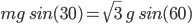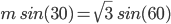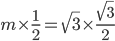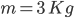Therefore value of mass m is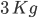.

## FAQs of Resultant Force

Question1. What is translational motion?

Solution: Motion in which all points of a moving body move uniformly in the same direction.

Question2. What is the difference between balanced and unbalanced forces?

Solution: Balanced forces do not cause any change in the state of motion of a body. Unbalanced forces accelerate the body.

Question3. What is Net Force ?

Solution: The vector sum of all the external forces that are acting on a system represents the net force on that object.

Question4. Which of the following is the condition for translational equilibrium?

Solution: Net force or resultant force on the object must be zero.

## NCERT Class 11 Physics Chapters

 Physical World Units and Measurements Motion in a Straight Line Motion in a Plane Laws of Motion Work Energy and Power Particles and Rotational Motion Gravitation Mechanical Properties of Solids Mechanical Properties in Liquids Thermal Properties of Matter Thermodynamics Kinetic Theory Oscillations WavesTalk to our expert
Resend OTP Timer =
By submitting up, I agree to receive all the Whatsapp communication on my registered number and Aakash terms and conditions and privacy policy